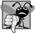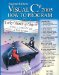# Indexers

Chapter 4 introduced properties as a way to access a class's private data in a controlled manner via the properties' get and set accessors. Sometimes a class encapsulates lists of data such as arrays. Such a class can use keyword this to define property-like class members called indexers that allow array-style indexed access to lists of elements. With "conventional" C# arrays, the index must be an integer value. A benefit of indexers is that you can define both integer indices and non-integer indices. For example, you could allow client code to manipulate data using strings as indices that represent the data items' names or descriptions. When manipulating "conventional" C# array elements, the array element access operator always returns a value of the same typei.e., the type of the array. Indexers are more flexiblethey can return any type, even one that is different from the type of the underlying data.

Although an indexer's element access operator is used like an array element access operator, indexers are defined like properties in a class. Unlike properties, for which you can choose an appropriate property name, indexers must be defined with keyword this. Indexers have the general form:

```accessModifier returnType this[ IndexType1 name1, IndexType2 name2, ... ]
{
get
{
// use name1, name2, ... here to get data
}
set
{
// use name1, name2, ... here to set data
}
}
```

The IndexType parameters specified in the brackets ([]) are accessible to the get and set accessors. These accessors define how to use the index (or indices) to retrieve or modify the appropriate data member. As with properties, the indexer's get accessor must return a value of type returnType and the set accessor can use the implicit parameter value to reference the value that should be assigned to the element.## Common Programming Error 9 3

Declaring indexers as static is a syntax error.

The application of Figs. 9.5 and 9.6 contains two classesclass Box represents a box with a length, a width and a height, and class BoxTest demonstrates class Box's indexers.

The private data members of class Box are string array names (line 6), which contains the names (i.e., "length", "width" and "height") for the dimensions of a Box, and double array dimensions (line 7), which contains the size of each dimension. Each element in array names corresponds to an element in array dimensions (e.g., dimensions[ 2 ] contains the height of the Box).

Box defines two indexers (lines 1833 and lines 3659) that each return a double value representing the size of the dimension specified by the indexer's parameter. Indexers can be overloaded like methods. The first indexer uses an int index to manipulate an element in the dimensions array. The second indexer uses a string index representing the name of the dimension to manipulate an element in the dimensions array. Each indexer returns -1 if its get accessor encounters an invalid subscript. Each indexer's set accessor assigns value to the appropriate element of dimensions only if the index is valid. Normally, you would have an indexer throw an exception if it receives an invalid index. We discuss how to throw exceptions in Chapter 12, Exception Handling.

Notice that the string indexer uses a while structure to search for a matching string in the names array (lines 4244 and lines 5254). If a match is found, the indexer manipulates the corresponding element in array dimensions (lines 46 and 57).

Class BoxTest (Fig. 9.6) manipulates the private data members of class Box through Box's indexers. Local variable box is declared at line 10, and initialized to a new instance of class Box. We use the Box constructor to initialize box with dimensions of 30, 30, and 30. Lines 1416 use the indexer declared with parameter int to obtain the three dimensions of box, and display them with WriteLine. The expression box[ 0 ] (line 14) implicitly calls the get accessor of the indexer to obtain the value of box's private instance variable dimensions[ 0 ]. Similarly, the assignment to box[ 0 ] in line 20 implicitly calls the set accessor in lines 2832 of Fig. 9.5. The set accessor implicitly sets its value parameter to 10, then sets dimensions[ 0 ] to value (10). Lines 24 and 2830 in Fig. 9.6 take similar actions, using the overloaded indexer with a string parameter to manipulate the same data.

Figure 9.5. Box class definition represents a box with length, width and height dimensions with indexers.

 ``` 1 // Fig. 9.5: Box.cs 2 // Box class definition represents a box with length, 3 // width and height dimensions with indexers. 4 public class Box 5 { 6 private string[] names = { "length", "width", "height" }; 7 private double[] dimensions = new double[ 3 ]; 8 9 // constructor 10 public Box( double length, double width, double height ) 11 { 12 dimensions[ 0 ] = length; 13 dimensions[ 1 ] = width; 14 dimensions[ 2 ] = height; 15 } 16 17 // indexer to access dimensions by integer index number 18 public double this[ int index ] 19 { 20 get 21 { 22 // validate index to get 23 if ( ( index < 0 ) || ( index >= dimensions.Length ) ) 24 return -1; 25 else 26 return dimensions[ index ]; 27 } // end get 28 set 29 { ``` 30 if ( index >= 0 && index < dimensions.Length ) 31 dimensions[ index ] = value; 32 } // end set 33 } // end numeric indexer 34 35 // indexer to access dimensions by their string names 36 public double this[ string name ] 37 { 38 get 39 { 40 // locate element to get 41 int i = 0; 42 while ( ( i < names.Length ) && 43 ( name.ToLower() != names[ i ] ) ) 44 i++; 45 46 return ( i == names.Length ) ? -1 : dimensions[ i ]; 47 } // end get 48 set 49 { 50 // locate element to set 51 int i = 0; 52 while ( ( i < names.Length ) && 53 ( name.ToLower() != names[ i ] ) ) 54 i++; 55 56 if ( i != names.Length ) 57 dimensions[ i ] = value; 58 } // end set 59 } // end string indexer 60 } // end class Box

``` 1 // Fig. 9.6: BoxTest.cs
3 using System;
4
5 public class BoxTest
6 {
7 public static void Main( string[] args )
8 {
9 // create a box
10 Box box = new Box( 30, 30, 30 );
11
12 // show dimensions with numeric indexers
13 Console.WriteLine( "Created a box with the dimensions:" );
14 Console.WriteLine( "box[ 0 ] = {0}", box[ 0 ] );
15 Console.WriteLine( "box[ 1 ] = {0}", box[ 1 ] );
16 Console.WriteLine( "box[ 2 ] = {0}", box[ 2 ] );
17
```
18 // set a dimension with the numeric indexer 19 Console.WriteLine( " Setting box[ 0 ] to 10... " ); 20 box[ 0 ] = 10; 21 22 // set a dimension with the string indexer 23 Console.WriteLine( "Setting box[ "width" ] to 20... " ); 24 box[ "width" ] = 20; 25 26 // show dimensions with string indexers 27 Console.WriteLine( "Now the box has the dimensions:" ); 28 Console.WriteLine( "box[ "length" ] = {0}", box[ "length" ] ); 29 Console.WriteLine( "box[ "width" ] = {0}", box[ "width" ] ); 30 Console.WriteLine( "box[ "height" ] = {0}", box[ "height" ] ); 31 } // end method Main 32 } // end class BoxTest
 ``` Created a box with the dimensions: box[ 0 ] = 30 box[ 1 ] = 30 box[ 2 ] = 30 Setting box[ 0 ] to 10... Setting box[ "width" ] to 20... Now the box has the dimensions: box[ "length" ] = 10 box[ "width" ] = 20 box[ "height" ] = 30 ```Visual C# 2005 How to Program (2nd Edition)
ISBN: 0131525239
EAN: 2147483647
Year: 2004
Pages: 600